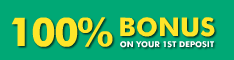# Live Sports Odds

The sub pages of this menu item contain live sports odds for different online sport book sites. Currently we're still fine tuning the pages. Soon you will be able to display the odds in your preferred format (decimal or fractional, see below), as well compare games at the different sport books against each other.

Odds are presented using either the European format (decimal odds) or the American format (fractional odds). Below is a useful Excel formula for converting the odds from one format to another.

## Fractional -> Decimal

IF(A1<0;ROUND(1+100/ABS(A1);2);(A1+100)/100)

## Decimal -> Fractional

IF(A2<2;-ROUND(1/(A2-1)*100;0);IF(A2=2;”Even”;A2*100-100))

The odds are converted so, that if there’s a plus (+) in front of the American odd, the odd is divided by a hundred (100), and 1 is added to the fraction, for example: +120=1+(120/100)=2.20. If there’s a minus (-) in front, the 100 is divided by this odd and 1 is added to the fraction, for example: -120=1+(100/120)=1.83.

Source: Wikipedia Sportsbook

### Recommended Sports Betting Site: Sportsbook